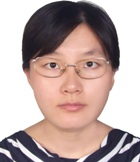## Associate prof. Liwei ShiAssociate professor, China University of Political Science and Law, Beijing, China. Main research interests: Numerical methods for partial differential equations; Numerical simulations of interface problems and their application; Numerical simulations of microfluidic chips. Teaching: Advanced Mathematics; Probability and Statistics; Advanced Mathematics for Liberal Arts Students. Research summary: Solving two/three dimensional elliptic/elasticity/parabolic partial differential equations with matrix-valued coefficient and sharp-edged interfaces using non-traditional finite element method; Numerical simulation and mathematical computation of ELISA (Enzyme Linked ImmunoSorbent Assay) test based on thermoelectric microfluidic chips.